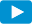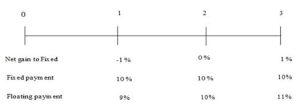Notes for Level I CFA® Program

R57 Basics of Derivative Pricing and Valuation

3.3. Pricing and Valuation of Swap ContractsWatch VideoTo understand how swaps work, let us consider a 3-year plain vanilla interest rate swap with annual settlement where the fixed-rate payer pays 10% and the floating rate payer pays an interest based on LIBOR. LIBOR rates at t = 0, 1 and 2 are 9%, 10% and 11%.Interpretation:

• At times 0, 1 and 2, the two parties exchange a series of payments. The fixed rate payer makes a fixed payment of 10% and receives a floating payment based on the value of the underlying at that point in time. Here is it 9%, 10% and 11% respectively.
• Like a forward rate agreement, rates are locked in for future. So, a swap is similar to a series of forward contracts, with each contract expiring at specific times, where one party agrees to make a fixed payment and receive a variable payment. But, the prices of the implicit forward contracts embedded in a swap are not equal.
• It is like getting into multiple forward rate agreements to lock in rates for different periods in the future at a different forward price.

Swap is a series of off-market forward contracts where:

• Each forward contract is created at a price and maturity equal to the fixed price of the swap with the same maturity and payment dates respectively.
• This means that the series of FRAs built into a swap are all off-market FRAs – some with positive values and some with negative values.
• The combined value of the off-market FRAs is zero.

Now, how do we determine the price of a swap that would result in a combined value of its FRAs to be zero? This is discussed in the following section.

Difference between Price and Value of a Swap Contract

Some key points regarding the price and value of a swap or as follows:

• The value of a swap at initiation is typically equal to zero.
• The swap price is determined at initiation through a process known as replication. No-arbitrage pricing is the key to pricing a swap. Replication implies that the valuation of a swap price is the present value of all the net cash flow payments from the swap.
• The value of a swap changes during the life of the contract.

Example of a Swap

Consider a 3-year plain vanilla interest rate swap with annual settlement where the fixed-rate payer pays 10% and the floating-rate payer pays based on LIBOR. LIBOR rates at t = 0, 1, and 2 are 9%, 10% and 11%.

The fixed rate of the swap is referred to as its price. In this case, it is 10%. A swap can be replicated as follows:• Step 1: Buy a floating-rate bond or any asset that pays coupons of unknown value S0, S1, q….SN at times t = 1, 2 ..N.
• Step 2: Borrow money to purchase this floating rate bond (equivalent to issuing a fixed-rate bond); the payments for the money borrowed are equal fixed-payments of FS0 (t) at t=1, 2, …N. This must have the same cash flow as the swap.
• The rate at which the money for the floater was borrowed is the price of the swap. Given the no-arbitrage pricing, the fixed rate on the swap must be equal to the fixed rate at which the fixed-rate bond was issued in step 2.
• The value of the swap during the life of a swap is based on the present value of the expected future cash flows. The cash flows, floating payments in particular, are based on the market price of the underlying.